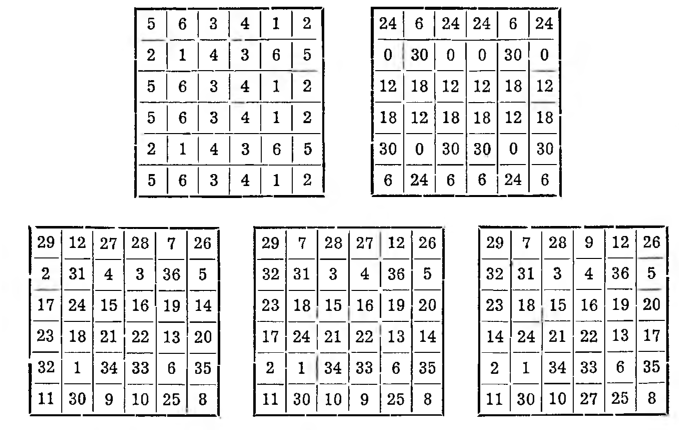# Construction of odd-even squares

Construction of odd-even squares

As we have already indicated, the fewest rules exist for the construction of this type of square, and all the methods are complicated and hard to remember. We quote the relatively easiest one here, whose creator is La Hire; but it too is far from being elegant, what characterizes the methods mentioned above. Proceeding similarly to even-even squares, we build auxiliary squares: the first of progress 1, 2, 3, 4, 5, 6, the second in progress 0, 6, 12, 18, 24, 30. Both of these squares are not magic, but their diagonals give a magic sum. When we sum these squares, we are also not getting the magic square yet.We can get it only after a series of adjustments, namely: leaving the diagonal figures in place, In the first row from the top and in the first left column we put numbers corresponding to each other 12 and 7, 27 and 28, 2 and 32, 17 and 23. In the second and last row, we switch: 4 and 3, 9 and 10. In the second and last column: 24 and 18, 14 and 20, A fourth will follow, the square given here, in which the numbers of the fourth row and the fourth column should also be changed: 17 and 14, 27 and 9. We will then get the fifth square, which is finally a magic square with a magic sum 111.

These displacements can be reduced to three general rules. Without touching the numbers standing on the diagonals, it changes in turn:

1° - in the first row and in the first column, the number of fields corresponding to each other;

2° - in the second and last row and in the second and last column - the number of middle squares;

3° - in one of the middle rows and - in one of the middle columns - the number of outermost boxes.

Of course, you can take the last row and the last column instead of the first row and the first column, it is also possible to modify the 2 ° and 3 ° adjustments, as long as you do not touch the numbers on the diagonal fields.

By building a magic square with auxiliary squares, you can plan, that there will be a certain number in a certain field, and it is relatively easy to do. For example, when we want, so that there is one in the middle field, then we start the construction of the first square from this field and put it there 1, and in the second square we strive for it, that it would fall out in that field 0.